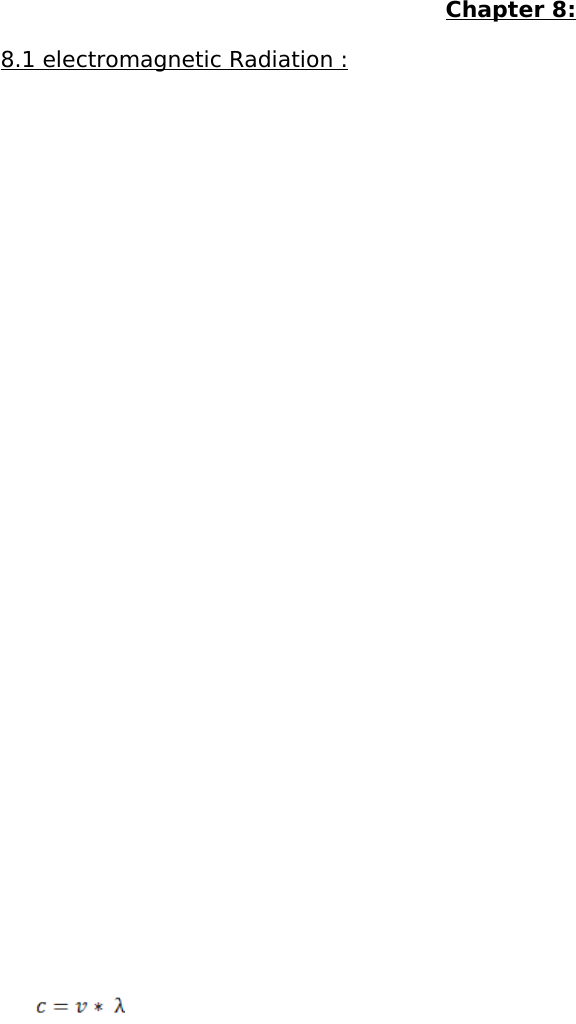Textbook Notes (280,000)
CA (160,000)
UTSC (20,000)
Chemistry (300)
CHMA10H3 (100)
Chapter 8

# Chapter 8

Department
Chemistry
Course Code
CHMA10H3
Professor
Ann Verner
Chapter
8

Page:
of 9Chapter 8:
Electromagnetic radiation: form of energy transmission in which electric and magnetic
fields are propagated as waves through empty space or a medium. – produced by
accelerating electrically charged particles ( ex. Radio waves, visible light )
- A wake is a disturbance that transmits energy through space or a
material medium
- Wave consist of crest, trough
Crest: high points where the rope is at the greatest height above the center line
Through: low points, where the rope is at the greatest dept below the center of the line
Amplitude: maximum height above or below the center of the line
Wavelength: distance between the top of the two successive crests (λ, m)
Frequency: # of crests or through that pass through a point in a given period of time ( ν,
Hz, S-1 - , # of events or cycles per sec)
- Product of λ and ν = speed ( how far the wave front travels in a unit of
time)
Electric field: region around electrically charged particle – detected by measuring the force
on an object when it is brought onto the field
Magnetic field: found in the region surrounding a magnet
Frequency, wavelength and speed of electromagnetic radiation:
- Speed of electromagnetic radiation ( its constant speed in a vacuum) :
3.00 * 108 S-1 (c)
- Wavelength for EMR is shorted for higher freq. And longer for lower freq.
An important characteristic of electromagnetic Waves:
When waves are said to be in:
- Phase: waves are in step upon meeting, their crests, troughs overlap, - waves
combine to produce highest crest, and deepest troughs -called constructive
interference
- Out of phase: “out of step” waves meet where the peek of one wave occurs at the
trough of another wave- waves cancel out , water is flat – destructive interference
Diffraction: dispersion of different of wavelength components of light beams through
a interference produced by reflection from a grooved service - property of waves
The visible spectrum:
- Waves of differing wavelengths have slightly differing speeds in air and other media
- when white light is passed through a transparent medium, wavelength
components are refracted differently
8.2 Atomic Spectra:
- visible spectrum said to be continuous spectrum because light being diffracted
consists of many wavelength components
- spectrum is said to be discontinuous if produced light have small number of wave
components
- discontinuous spectra are called: atomic or line spectra
- 5 lines in the spectrum of He that can be seen by the human eye
- In the He equation n has to be greater than 2
8.3 Quantum theory:
- Hot objects emit light of different colors
Black body radiation: light emission by heated solids
Quantum of energy: the diff between any 2 allowed energies of a system also have a
specific value
- Planck’s constant: h= 6.62607 * 10-34 Js
- Energy of a quantum of electromagnetic radiation (h) is proportional to the freq. Of
E=hv
The photoelectric effect:
Photoelectric effect: when light strikes the surface of a certain metal , e’ are ejected
- Electron emission only occurs when the freq exceeds a particular threshold ( v0)
When this condition is met:
1. # of e’ emmited depends on the intensity of the incident light
2. Kinetic energy of the emitted e’ depends on the freq of the light.
- Particles of light = photons
- Photons of energy (hv) strike a bound e’, which absorbs the photons energy
- If photons energy greater than the binding e’ to the surface a photoelectron is
librated
- Lowest freq light producing the photoelectric effect is the threshold freq. Any
energy in excess is appears as kinetic energy in the emitted photoelectrons
Vs=k(v-v0)
- Vs is proportional to the freq
- Constant k is independent of the metal used, v0 varies from one metal to another
- Photoelectric current is proportional to the light intensity (I)
- Photoelectrons increase with the intensity of light – associate light intensity with the
# of photons arriving at a point per unit of time
8.4 The bohr atom:
- e’ has only a fixed set of allowed orbits stationary states
- as long as an e’ remains in a given orbit, its energy is constant and no energy is
emitted
- angular momentum – discrete set of allowed orbits
- e’ can only pass from one allowed orbit to another
- the Bohr theory predicts the radii of allowed orbits in a H atom:
To find radius: Rn=n2a0 where n= 1,2,3... and a0 = 53 pm
- when the e’ is free from the nucleus it is said to be at zero energy
- when a free e’ is attracted to the nucleus and confined to the orbit n, the e’ energy
becomes negative RH= 2.179 * 10-18 J ( En energy of an orbit)
- n=1 ( for H) – lowest allowed energy ground state
- excited state: when e’ gains energy and moves to a higher level
- when an e’ drops from a higher to a lowered number orbital unique amount of
energy it emitted
The Bohr theory and spectroscopy:
- emission spectrum of a sample can be measured by dispersing emitted light
through a prism and determining the wavelengths of the individual component of
the light.
two types of spectroscopy: emission and absorption
-
-
- farther apart the energy levels the shorter the wavelength of the photons needed to
induce the transition